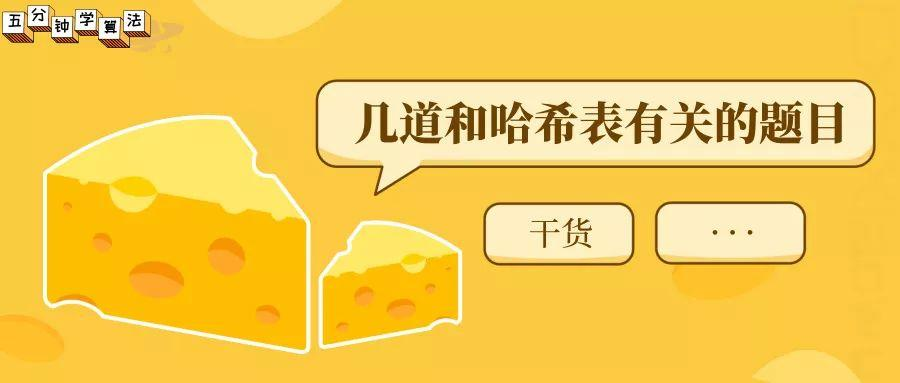## 1. 两数之和

### 题目描述

给定 nums = [2, 7, 11, 15], target = 9因为 nums + nums = 2 + 7 = 9所以返回 [0, 1]

### 题目解析

• 每次遍历时使用临时变量 complement 用来保存目标值与当前值的差值

• 在此次遍历中查找 record ，查看是否有与 complement 一致的值，如果查找成功则返回查找值的索引值与当前变量的值i

• 如果未找到，则在 record 保存该元素与索引值 i

### 代码实现

// 1. Two Sum// 时间复杂度：O(n)// 空间复杂度：O(n)class Solution {public:    vector<int> twoSum(vector<int>& nums, int target) {        unordered_map<int,int> record;        for(int i = 0 ; i < nums.size() ; i ++){            int complement = target - nums[i];            if(record.find(complement) != record.end()){                int res[] = {i, record[complement]};                return vector<int>(res, res + 2);            }            record[nums[i]] = i;        }    }};

## 2. 无重复字符的最长子串

### 代码实现

class Solution {public:    int lengthOfLongestSubstring(string s) {        int res = 0, left = -1, n = s.size();        unordered_map<int, int> m;        for (int i = 0; i < n; ++i) {            if (m.count(s[i]) && m[s[i]] > left) {                left = m[s[i]];              }            m[s[i]] = i;            res = max(res, i - left);                    }        return res;    }};

### 拓展

• （1）如果当前遍历到的字符从未出现过，那么直接扩大右边界；

• （2）如果当前遍历到的字符出现过，则缩小窗口（左边索引向右移动），然后继续观察当前遍历到的字符；

• （3）重复（1）（2），直到左边索引无法再移动；

• （4）维护一个结果 res，每次用出现过的窗口大小来更新结果 res ，最后返回 res 获取结果。

### 代码实现

// 3. Longest Substring Without Repeating Characters// 滑动窗口// 时间复杂度: O(len(s))// 空间复杂度: O(len(charset))class Solution {public:    int lengthOfLongestSubstring(string s) {        int freq = {0};        int l = 0, r = -1; //滑动窗口为s[l...r]        int res = 0;        // 整个循环从 l == 0; r == -1 这个空窗口开始        // 到l == s.size(); r == s.size()-1 这个空窗口截止        // 在每次循环里逐渐改变窗口, 维护freq, 并记录当前窗口中是否找到了一个新的最优值        while(l < s.size()){            if(r + 1 < s.size() && freq[s[r+1]] == 0){                r++;                freq[s[r]]++;            }else {   //r已经到头 || freq[s[r+1]] == 1                freq[s[l]]--;                l++;            }            res = max(res, r-l+1);        }        return res;    }};

## 3. 三数之和

### 代码实现

class Solution {public:    vector<vector<int>> threeSum(vector<int>& nums) {        vector<vector<int>> res;        sort(nums.begin(), nums.end());        if (nums.empty() || nums.back() < 0 || nums.front() > 0) return {};        for (int k = 0; k < nums.size(); ++k) {            if (nums[k] > 0) break;            if (k > 0 && nums[k] == nums[k - 1]) continue;            int target = 0 - nums[k];            int i = k + 1, j = nums.size() - 1;            while (i < j) {                if (nums[i] + nums[j] == target) {                    res.push_back({nums[k], nums[i], nums[j]});                    while (i < j && nums[i] == nums[i + 1]) ++i;                    while (i < j && nums[j] == nums[j - 1]) --j;                    ++i; --j;                } else if (nums[i] + nums[j] < target) ++i;                else --j;            }        }        return res;    }};

## 4. 重复的 DNA 序列

### 题目解析

A: 0100 0001　　C: 0100 0011　　G: 0100 0111　　T: 0101 0100

### 解题代码

class Solution {public:    vector<string> findRepeatedDnaSequences(string s) {        vector<string> res;        if (s.size() <= 10) return res;        int mask = 0x7ffffff, cur = 0;        unordered_map<int, int> m;        for (int i = 0; i < 9; ++i) {            cur = (cur << 3) | (s[i] & 7);        }        for (int i = 9; i < s.size(); ++i) {            cur = ((cur & mask) << 3) | (s[i] & 7);            if (m.count(cur)) {                if (m[cur] == 1) res.push_back(s.substr(i - 9, 10));                ++m[cur];             } else {                m[cur] = 1;            }        }        return res;    }};

## 5. 两个数组的交集

### 题目解析

• 遍历 num1，通过 set 容器 record 存储 num1 的元素

• 遍历 num2，在 record 中查找是否有相同的元素，如果有，用 set 容器 resultSet 进行存储

• 将 resultSet 转换为 vector 类型

### 代码实现

// 时间复杂度: O(nlogn)// 空间复杂度: O(n)class Solution {public:    vector<int> intersection(vector<int>& nums1, vector<int>& nums2) {        set<int> record;        for( int i = 0 ; i < nums1.size() ; i ++ ){            record.insert(nums1[i]);        }        set<int> resultSet;        for( int i = 0 ; i < nums2.size() ; i ++ ){            if(record.find(nums2[i]) != record.end()){                resultSet.insert(nums2[i]);            }        }        vector<int> resultVector;        for(set<int>::iterator iter = resultSet.begin(); iter != resultSet.end(); iter ++ ){            resultVector.push_back(*iter);        }        return resultVector;    }};

## 6. 两个数组的交集 II

### 题目描述

输入: nums1 = [1,2,2,1], nums2 = [2,2]输出: [2,2]

输入: nums1 = [4,9,5], nums2 = [9,4,9,8,4]输出: [4,9]

### 题目解析

• 遍历 num1，通过 map 容器 record 存储 num1 的元素与频率；

• 遍历 num2 ，在 record 中查找是否有相同的元素（该元素的存储频率大于 0 ），如果有，用 map 容器resultVector 进行存储，同时该元素的频率减一。

### 代码实现

// 时间复杂度: O(nlogn)// 空间复杂度: O(n)class Solution {public:    vector<int> intersect(vector<int>& nums1, vector<int>& nums2) {        map<int, int> record;        for(int i = 0 ; i < nums1.size() ; i ++){             record[nums1[i]] += 1;        }        vector<int> resultVector;        for(int i = 0 ; i < nums2.size() ; i ++){            if(record[nums2[i]] > 0){                resultVector.push_back(nums2[i]);                record[nums2[i]] --;            }        }        return resultVector;    }};

## 7. 回旋镖的数量

### 题目解析

n 最大为 500，可以使用时间复杂度为 O(n^2)的算法。

• 遍历所有的点，让每个点作为一个锚点

• 然后再遍历其他的点，统计和锚点距离相等的点有多少个

• 然后分别带入 n(n-1) 计算结果并累加到 res 中

• 如果有一个点a，还有两个点 b 和 c ，如果 ab 和 ac 之间的距离相等，那么就有两种排列方法 abc 和 acb ；

• 如果有 n 个点和点 a 距离相等，那么排列方式为 n(n-1)；

• 计算距离时不进行开根运算, 以保证精度；

• 只有当 n 大于等于 2 时，res 值才会真正增加，因为当n=1时，增加量为1 * ( 1 - 1 ) = 0

### 代码实现

// 时间复杂度: O(n^2)// 空间复杂度: O(n)class Solution {public:    int numberOfBoomerangs(vector<pair<int, int>>& points) {        int res = 0;        for( int i = 0 ; i < points.size() ; i ++ ){            // record中存储 点i 到所有其他点的距离出现的频次            unordered_map<int, int> record;            for(int j = 0 ; j < points.size() ; j ++){                if(j != i){                    // 计算距离时不进行开根运算, 以保证精度                    record[dis(points[i], points[j])] += 1;                }            }            for(unordered_map<int, int>::iterator iter = record.begin() ; iter != record.end() ; iter ++){                res += (iter->second) * (iter->second - 1);            }        }        return res;    }private:    int dis(const pair<int,int> &pa, const pair<int,int> &pb){        return (pa.first - pb.first) * (pa.first - pb.first) +               (pa.second - pb.second) * (pa.second - pb.second);    }};

## 8. 四数相加 II

### 题目解析

Two Sum 极其类似，使用哈希表来解决问题。

• 把 A 和 B 的两两之和都求出来，在哈希表中建立两数之和与其出现次数之间的映射；

• 遍历 C 和 D 中任意两个数之和，只要看哈希表存不存在这两数之和的相反数就行了。

### 代码实现

// 时间复杂度: O(n^2)// 空间复杂度: O(n^2)class Solution {public:    int fourSumCount(vector<int>& A, vector<int>& B, vector<int>& C, vector<int>& D) {        unordered_map<int,int> hashtable;        for(int i = 0 ; i < A.size() ; i ++){            for(int j = 0 ; j < B.size() ; j ++){                 hashtable[A[i]+B[j]] += 1;            }        }        int res = 0;        for(int i = 0 ; i < C.size() ; i ++){            for(int j = 0 ; j < D.size() ; j ++){                if(hashtable.find(-C[i]-D[j]) != hashtable.end()){                    res += hashtable[-C[i]-D[j]];                }            }        }        return res;    }};

## 链表算法面试问题？看我就够了！